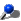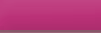﻿ Virtual Commodities News Bulletin (VCNB) commodity futures traders webtrading information guideinquire about domain

# VCNB.COM --- The Virtual Commodities News Bulletin

## "How to Profitably Trade Commodity Futures"

### Commodity Futures Trading Success by using Fibonacci Numbers

A good time to study and use Fibonacci techniques in your commodity futures and stock trading is to start today so learn as much as possible about Fibonacci Numbers as fast as you can. Although the commodity futures and fx forex markets actually comply to 'natural law' or it is simply the result of many commodity futures trading programs acting in unison with each other thus the markets tend to react to a certain set of ratios. Before we go into what these ratios are, we'll first cover where they are derived from.

Back in the late 12th century lived a man named Leonardo Fibonacci de Pisa. It is said when he had returned from a trip to Egypt he somehow ran across a set of numbers with somewhat unique and many remarkable properties. Apparently, it was via his studies of the Great Pyramid in Gizeh Egypt he observed the "Golden Ratio" the ancient Egyptians had integrated into the Pyramid ratios, size and its dimensions. Today we refer to this famous sequence of numbers as "Fibonacci Numbers."

The Fibonacci numbering sequence follows: 1, 1, 2, 3, 5, 8, 13, 21, 34, 55, 89, 144, 233, 377… As you can see, each number is the result of adding together the prior 2 numbers in the fib sequence. This can be repeated into infinity.

The interesting properties of these numbers, after getting past the first 4, are as follows: Each number in the sequence is approximately 1.618 the previous number. The higher the number, the closer to this ratio it will be. On the reverse, each number is approximately .618 of the next higher number.

The higher the number, the closer to this ratio it will be. Alternate numbers will be 2.618 of each other, such as 377 to 144. The inverse is .382.These ratios also interrelate to each other as follows:

• Square of .618 = .382.
• Square of 1.618 = 2.618
• 2.618 x .382 = 1
• 2.618 – 1.618 = 1
• 1.618 x .618 = 1
• 1.618 - .618 = 1

There are many other interesting facts that we can bring up about the Fibonacci numbers and ratios, but we'll dispense with it now and discuss the application of Fibonacci to market analysis and trading.

Fibonacci ratios more often than not manifest itself in the patterns of market prices. By examining any price chart, you should be able to note this for yourself.The simple approach to using Fibonacci is in price support - resistance discovery.

As found in many commodity trading programs today,what is called 'Fibonacci Retracements' are derived by taking a market range, such as a market bottom to a top, and dividing that range by these Fibonacci ratios of .382, .618.

There are also other Fibonacci ratios you can use, such as .786 and .236. .786 is the square root of .618, and .236 is from multiplying .382 with .618. Example: Suppose we look on our daily price chart and see an obvious market bottom at 150. Now suppose that price moved up for some days and eventually made a top at 200.

We know this to be a top because price has started to move down from that top. All we would have to do to get our Fibonacci static support and resistance level prices is to take the top price of 200 and subtract from that the bottom price of 150. Our RANGE would then be 50-points.

Take the RANGE (50-Points) and multiply it by the Fibonacci ratios of .236, .382, .618 and .786. We would end up with the following results:

(50 x .236) = 11.8
(50 x .382) = 19.1
(50 x .618) = 30.9
(50 x .786) = 39.3

Since price is moving down from the top price of 200, we would subtract the results of these ratios from 200 to get our SUPPORT prices.

(200 – 11.8) = 188.20
(200 – 19.1) = 180.90
(200 – 30.9) = 169.10
(200 – 39.3) = 160.70

The process is the same for price ranges that go from top to bottom, where price is starting to move up again. In those cases you would simply add the results of those ratios to the bottom price to get your RESISTANCE prices.

Fibonacci ratios can also be useful at times for discovering when a top or bottom may form in the commodity you are trading. The process is to simply count the days between two tops or bottoms (or whatever combination you care for) and multiply by .618. Take the result and add to the second top or bottom in your equation to forecast out a possible turn.

Another time approach is to take any major top or bottom and then count forward a Fibonacci number of days into the future, such as 34, 55, 89 days and so-forth, that will produce a future date in which to look for a possible market top or bottom to occur.

As with anything else in trading, price will not always find Fibonacci support / resistance nor will it always turn at your Fibonacci time day counts. Other methods and indicators could and should be employed along with Fibonacci ratio work in market analysis. However, due to the frequency of ratios affecting market action, it would be worth your time to further your study in Fibonacci numbers/ratios. To read more about successfully commodity trading click-here!

#### Recommended Websites of InterestClick now for Trading Tip of the DayW.D. Gann Trading CoursesOption Trading CourseNATURE'S LAW Secret of the UniverseMicro Forex FuturesCommodity Trading SystemCorn Trading SystemOccam's RazorForex SystemToday's Date and Time